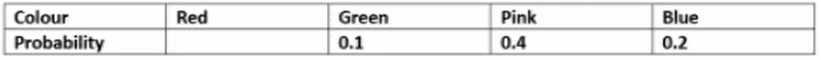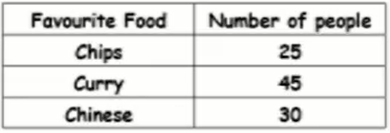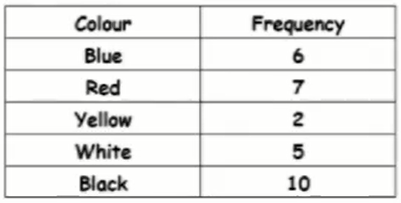If the spinner is spun 700 times, how many times would you expect it to land on red?Work out the probability that the spinner will land on red.How many people would you expect to like curry if 1000 people were asked?How many people would you expect to like curry if 400 people were asked?What is the probability the person didn't like curry best?What is the probability the person liked chips?How many white cars would you expect if 240 cars went past?How many white cars would you expect if 60 cars went past?What is the probability the next car will be not black?What is the probability the next car will be red?What is the probability the next car will be blue?